# 【数据结构与算法】-（8.3）KMP算法

【数据结构与算法】-（1）基础篇

【数据结构与算法】-（2）线性表基础

【数据结构与算法】-（3）循环链表（单向）

【数据结构与算法】-（4）双向链表和双向循环链表

【数据结构与算法】-（5）链表面试题解析

【数据结构与算法】-（6）栈

【数据结构与算法】-（7）队列

【数据结构与算法】-（8）栈之算法题

【数据结构与算法】-（8.1）字符串去重算法

【数据结构与算法】-（8.2）字符串搜索算法和RK&BP算法

## 二、原理

### 2.1 回顾BF 算法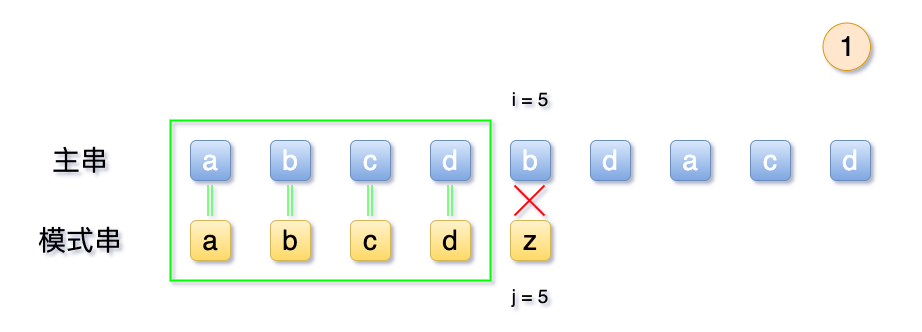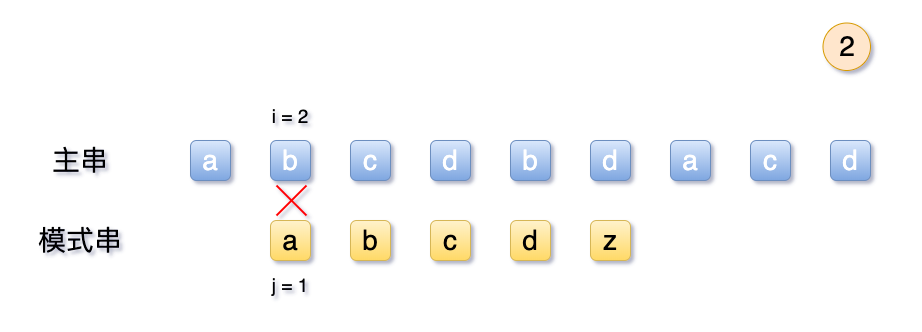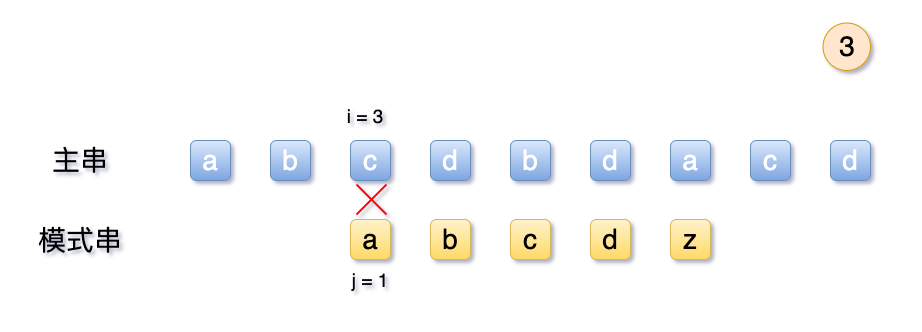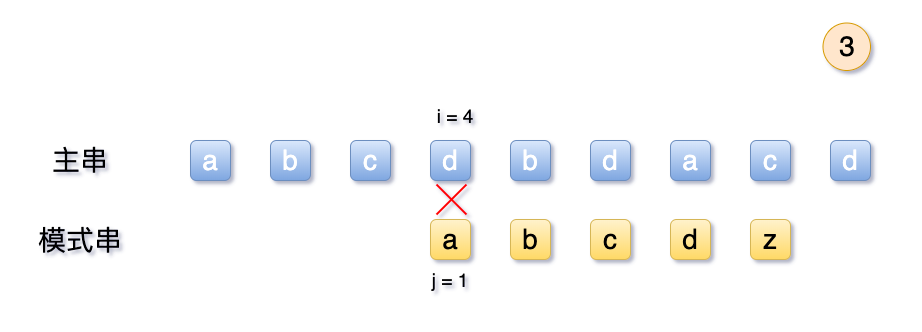### 2.3 场景A：模式串`T` 无重复

`S``T`模式串 `T` 中前4位均匹配，第5位匹配失败。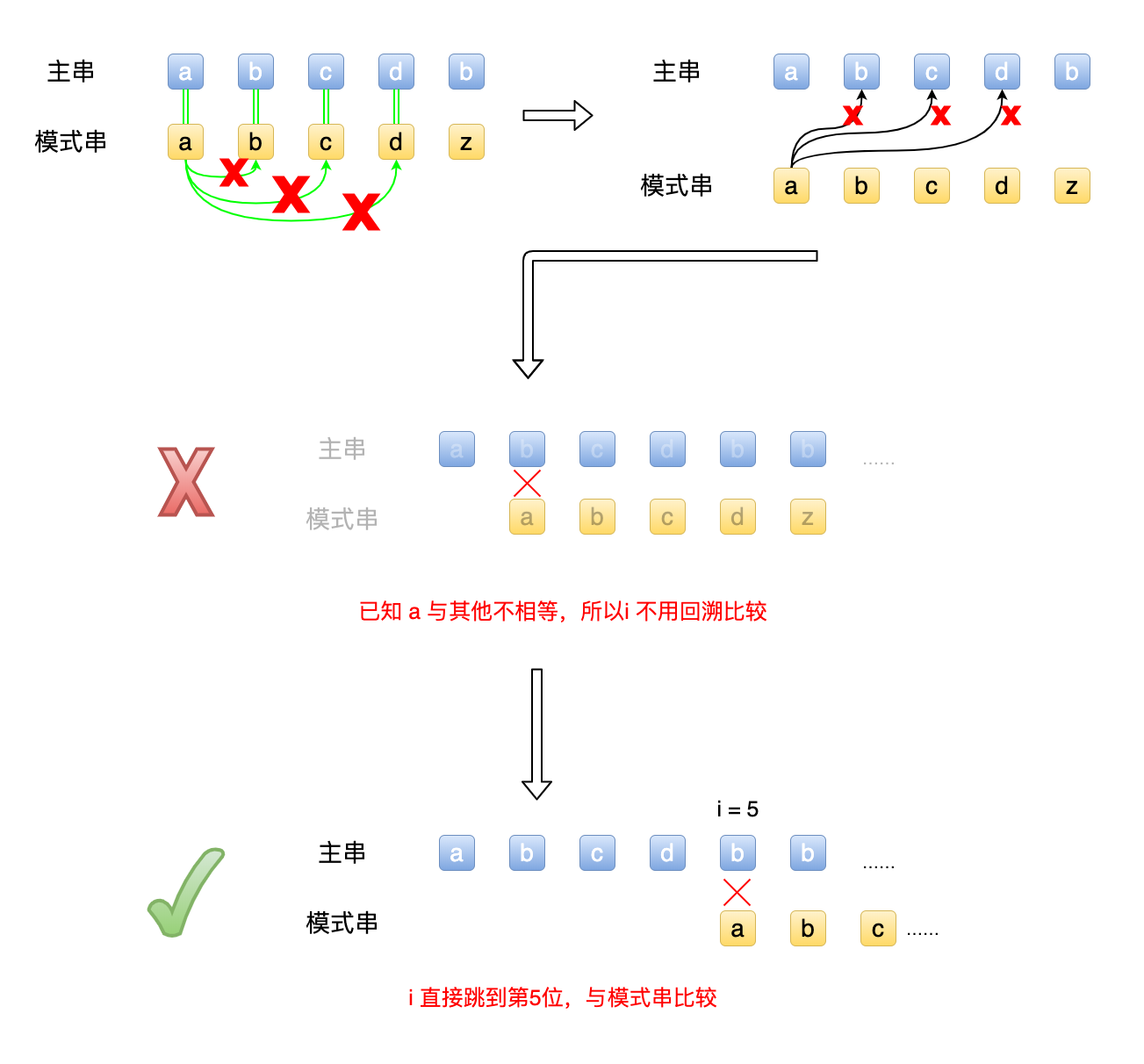### 2.4 场景B：模式串`T`有重复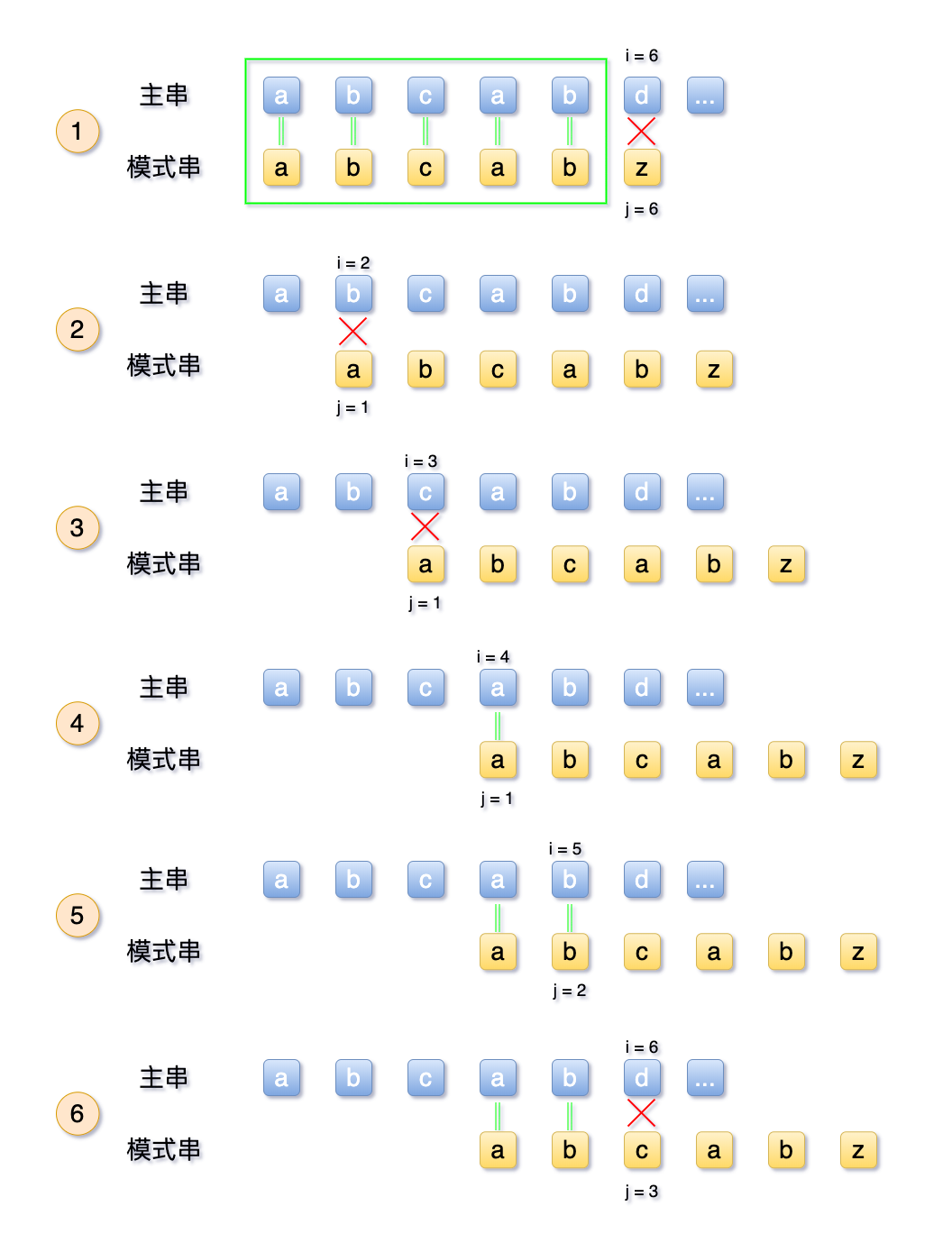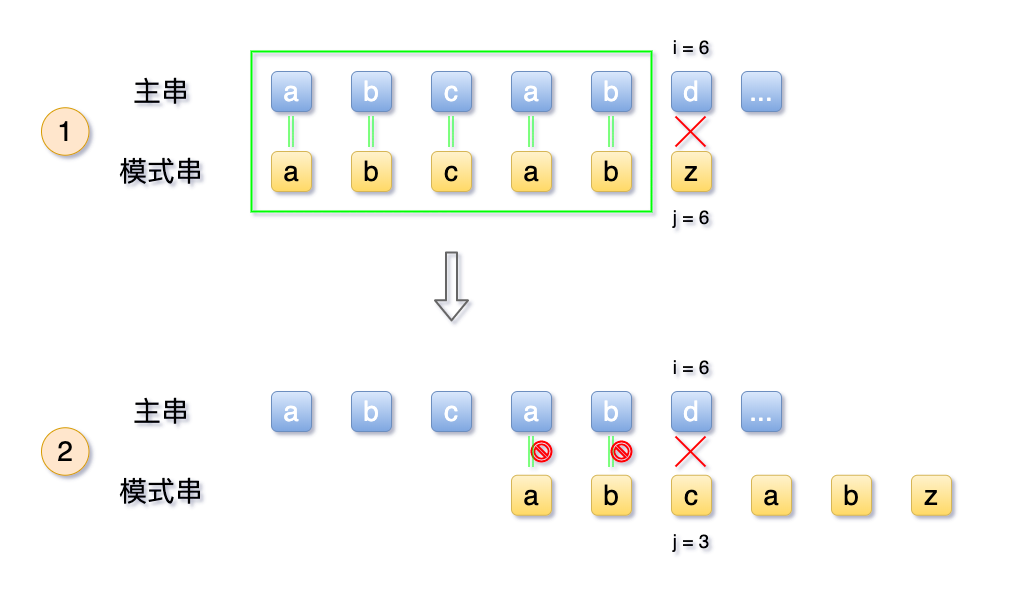### 2.5 思考归纳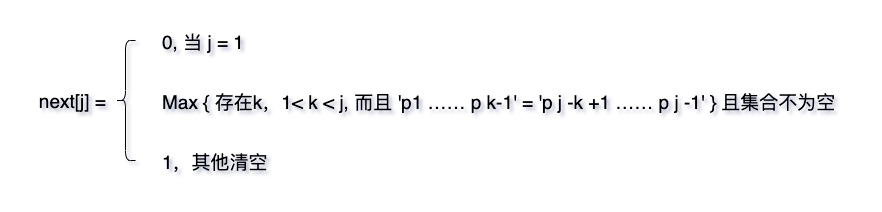### 2.5 逆推next 数组

#### 2.5.1 模式串为：abcdef

j 123456

1. 当 j = 1 时，next[j] = 1 ，属第一种情况
2. 当 j = 2 时，此时 j 从1位（a）到 j - 1 （b）位中，只有一个字符 ‘a’， 属于其他情况，此时 next = 1
3. 当 j = 3 时，此时 j 从1位（a）到 j - 1 （ab）位中，有2个字符 ‘ab’，但是a、b并不相等，第二种情况并不满足，所以此时 next = 1
4. 当 j = 4 时，此时 j 从1位（a）到 j - 1 （ab）位中，有3个字符 ‘abc’，但是a、b、c并不相等，第二种情况并不满足，所以此时 next = 1
5. 当 j = 5 时，此时 j 从1位（a）到 j - 1 （abcde）位中，有4个字符 ‘abcd’，但是a、b、c、d并不相等，第二种情况并不满足，所以此时 next = 1
6. 于是总结一下得出next 数组为 01111
j 12345

next[j] 01111

#### 2.5.2 模式串为：abcabf

j 123456

1. 当 j = 1 时，next[j] = 1 ，属第一种情况
2. 当 j = 2 时，此时 j 从1位（a）到 j - 1 （b）位中，只有一个字符 ‘a’， 属于其他情况，此时 next = 1
3. 当 j = 3 时，此时 j 从1位（a）到 j - 1 （ab）位中，有2个字符 ‘ab’，但是a、b并不相等，第二种情况并不满足，所以此时 next = 1
4. 当 j = 4 时，此时 j 从1位（a）到 j - 1 （ab）位中，有3个字符 ‘abc’，但是a、b、c并不相等，第二种情况并不满足，所以此时 next = 1
5. 当 j = 5 时，此时 j 从1位（a）到 j - 1 （abcde）位中，有4个字符 ‘abcd’，第1位、第4位的a相等，所以此时k = 5，即next = 2
6. 当 j = 6 时，此时 j 从1位（a）到 j - 1 （abcde）位中，有4个字符 ‘abcd’，第1位、第4位的a相等，所以此时k = 5，即next = 2
7. 于是总结一下得出next 数组为 011123
j 123456

next[j] 011123

#### 2.5.3 模式串为：abcbbabc

j 12345678

1. 当 j = 1 时，next[j] = 1 ，属第一种情况
2. 当 j = 2 时，此时 j 从1位（a）到 j - 1 （b）位中，只有一个字符 ‘a’， 属于其他情况，此时 next = 1
3. 当 j = 3 时，此时 j 从1位（a）到 j - 1 （ab）位中，有2个字符 ‘ab’，但是a、b并不相等，第二种情况并不满足，所以此时 next = 1
4. 当 j = 4 时，此时 j 从1位（a）到 j - 1 （ab）位中，有3个字符 ‘abc’，但是a、b、c并不相等，第二种情况并不满足，所以此时 next = 1
5. 当 j = 5 时，此时 j 从1位（a）到 j - 1 （abcb）位中，有4个字符 ‘abcb’，虽然第2、第4相等，但是并不满足前缀相等，所以此时k = 5，即next = 1
6. 当 j = 6 时，此时 j 从1位（a）到 j - 1 （abcba）位中，abcba，首位相等，取第一位 + 1，所以此时k = 2，即next] = 2
7. 当 j = 7 时，此时 j 从1位（a）到 j - 1 （abcbab）位中，abcbab，首位相等，取第2位 + 1，所以此时k = 3，即next = 3
8. 当 j = 8 时，此时 j 从1位（a）到 j - 1 （abcbab）位中，abcbab，首位相等，取第3位 + 1，所以此时k = 4，即next = 4

j 12345678

next[j] 01111234

#### 2.5.4 模式串为：aaaaab

j 123456

1. 当 j = 1 时，next[j] = 1 ，属第一种情况
2. 当 j = 2 时，当 j = 2 时，此时 j 从1位（a）到 j - 1 （b）位中，只有一个字符 ‘a’， 属于其他情况，此时 next = 1
3. 当 j = 3 时，此时 j 从1位（a）到 j - 1 （aa）位中，有aa 相等，取前面出现的序号+1，得k = 1+1=2，即 next = 2
4. 当 j = 4 时，此时 j 从1位（a）到 j - 1 （aaa）位中，前缀为 aa，后缀也为 aa， 取前面出现的序号+1，得k = 2+1=2，即 next = 3
5. 当 j = 5 时，此时 j 从1位（a）到 j - 1 （aaaa）位中，有aaa ，后缀 aaa，后缀也是 aaa 取前面出现的序号+1，得k = 3+1=4，即 next = 4
6. 当 j = 6 时，此时 j 从1位（a）到 j - 1 （aaaaa）位中，有aaaaa ，后缀 aaaa，后缀也是 aaa 取前面出现的序号+1，得k = 4+1=4，即 next = 5

j 123456

next[j] 012345

## 三、代码实现

### 3.1 获取next 数组

``````typedef char String[MAXSIZE+1]; /*  0号单元存放串的长度 */

void get_nextArray(String T, int *next)
{
int i, j;
i = 1;
j = 0;
next = 0;        // 这是第一种情况，初始为0

while ( i < T)     // 循环上限为模式串的长度
{
// 比较模式串 T 的前缀后缀俩字符,
//若相等，分别递增
if( j == 0 || T[i] == T[j])
{
++i;
++j;
next[i] = j;
}
else
{
// 如果不相等， j 回溯回当前
j = next[j]    ;
}
}
}``````

### 3.2 获取匹配的字符串

``````int getIndexKMP(String S, String T, int pos)
{
// s         = 主串
// T         = 模式串
// pos     = 最终符合需求的位置
int i = pos;
int j = 1;

// next 数组的初始化
int next;

// 根据模式串，定义出next
get_nextArray(T, next);

// 开始判断
while (i <= S && j <= T)
{
// 主串与字串如果相等，继续
if (j == 0 || S[i] == T[j])
{
++i;
++j;
}else{

// 两者不相等， j 回溯，具体位置为数组中的值
j = next[j];
}
}

// 如果 j 的长度大于模式串长度，即已经取得成功
if (j > T)
return i - T;
else
return 0;
}``````

### 3.3 运行检验

IDE 里操作一下，奥利给！

``````int main(int argc, const char * argv[]) {
// insert code here...

printf("第一次查询");
String S, T;
StrAssign(S, "cccaababcabc");
StrAssign(T, "abc");
int result = getIndexKMP(S, T, 1);
if (result > 0) {
printf("在里面的序号为:     %d\n\n", result);
}else{
printf("查找失败，主串并无匹配的模式串!\n\n");
}
return 0;
}``````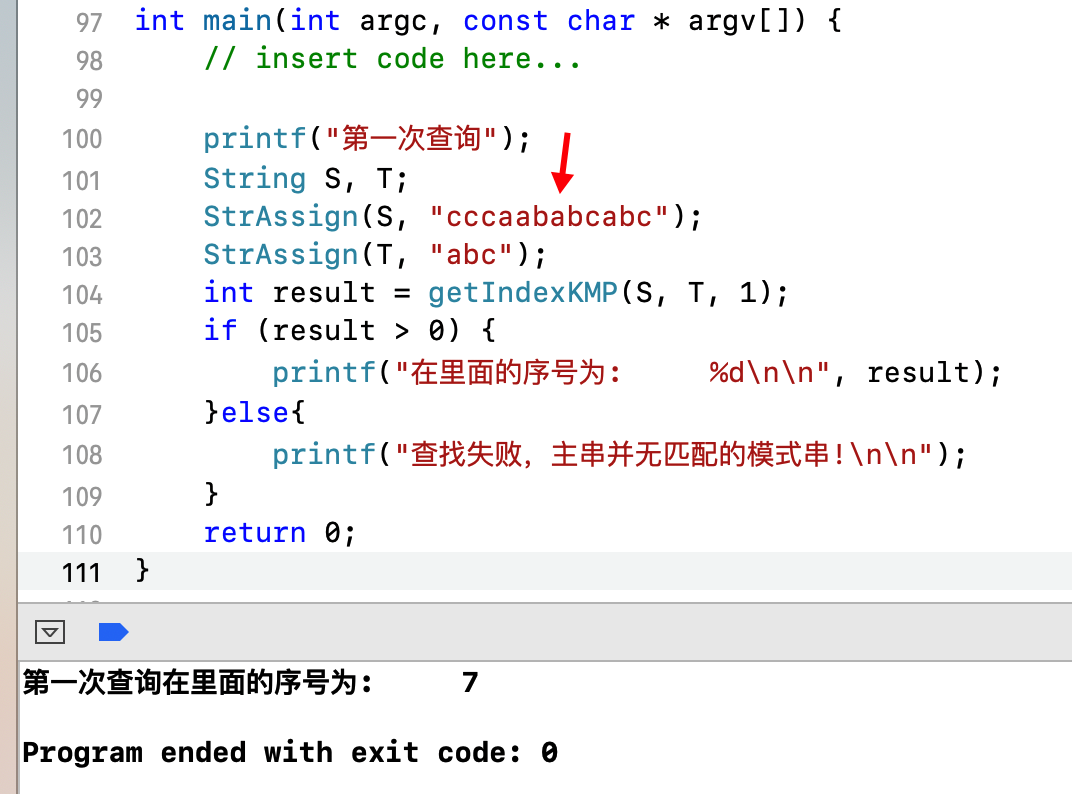## 四、优化改进

### 4.1 分析不足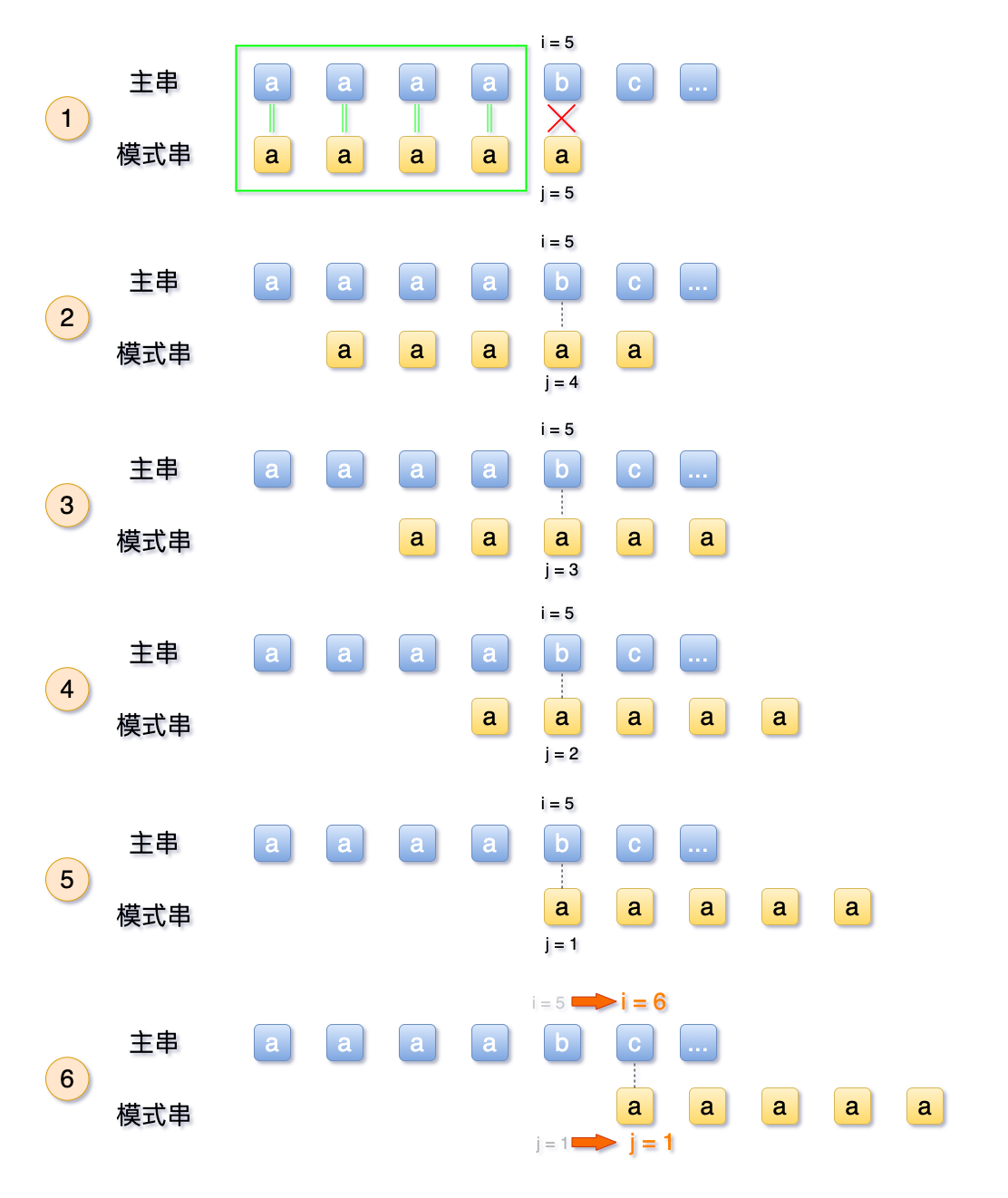### 4.2 新的nextVal 数组

``````void get_nextVal(String T, int *nextVal)
{
int i, j;
i = 1;
j = 0;
nextVal = 0;        // 这是第一种情况，初始为0

while ( i < T)     // 循环上限为模式串的长度
{
// 比较模式串 T 的前缀后缀俩字符,
//若相等，分别递增
if( j == 0 || T[i] == T[j])
{
++i;
++j;
if (T[i] == T[j]) {
nextVal[i] =  nextVal[j];
}else{
nextVal[i] = j;
}
}
else
{
// 如果不相等， j 回溯回当前
j = nextVal[j] ;
}
}
}``````

``````if (T[i] == T[j])
nextVal[i] = nextVal[j];``````

### 4.3逆推nextVal数组

#### 4.3.1 模式串为：ababaaaba

j 123456789

next[j] 011234223
nextVal[j] 010104210
1. j = 1, nextVal = 0;
2. j = 2, 照旧。左边为T  = b，右边 T[next] = T = a 这种前后字符不相等，还是沿用之前next[i] 的逻辑，即 = 1；
3. j = 3，此时为新情况，左边为T  = a，右边 T[next] = T = a 这种前后字符相等，使用新逻辑：nextVal = nextVal = 0；
4. j = 4 ，此时为新情况，左边为T  = b，右边 T[next] = T = b 这种前后字符相等，使用新逻辑：nextVal = nextVal = 1；
5. j = 5，此时为新情况，左边为T  = a，右边 T[next] = T = a 这种前后字符相等，使用新逻辑：nextVal = nextVal = 0；
6. j = 6，照旧。左边为T  = a，右边 T[next] = T = b 这种前后字符不相等，还是沿用之前next[i] 的逻辑，即 = 4；
7. j = 7，照旧。左边为T  = a，右边 T[next] = T = b 这种前后字符不相等，还是沿用之前next[i] 的逻辑，即 = 2；
8. j = 8，此时为新情况，左边为T  = b，右边 T[next] = T = b 这种前后字符相等，使用新逻辑：nextVal = nextVal = 1；
9. j = 9，此时为新情况，左边为T  = a，右边 T[next] = T = a 这种前后字符相等，使用新逻辑：nextVal = nextVal = 0；

#### 4.3.2 模式串为：aaaacd

j 123456

next[j] 012345
nextValue[j] 000005

1. j = 1, nextVal = 0;

2. j = 2, 此时为新情况，左边为T  = a，右边 T[next] = T = a 这种前后字符相等，使用新逻辑：nextVal = nextVal = 0；

3. j = 3，此时为新情况，左边为T  = a，右边 T[next] = T = a 这种前后字符相等，使用新逻辑：nextVal = nextVal = 0；

4. 同上累加

5. 同上累加

6. 同上累加

7. j = 6，此时照旧。左边T = d， 右边 T [next] = T  = a， 两者并不相等，沿用之前的next，即 nextVal = 5

### 4.4 改进小结

``if (T[i] == T[j]) { nextVal[i] = nextVal[j];}``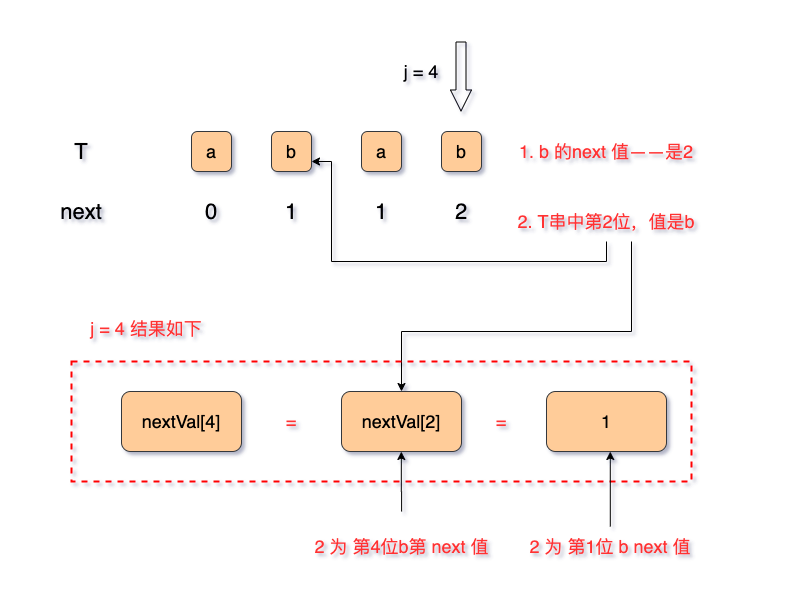上一篇【数据结构与算法】-（9）二叉树与顺序表实现
【数据结构与算法】-（1）基础篇 【数据结构与算法】-（2）线性表基础 【数据结构与算法】-（3）循环链表（单向） 【数据结构与算法】-（4）双向链表和双向循环链表 【数据结构与算法】-（5）链表面试题解析 【数据结构与算法】-（6）栈
2020-04-26 李佳【数据结构与算法】-（8.2）字符串搜索算法和RK&BP算法
【数据结构与算法】-（1）基础篇 【数据结构与算法】-（2）线性表基础 【数据结构与算法】-（3）循环链表（单向） 【数据结构与算法】-（4）双向链表和双向循环链表 【数据结构与算法】-（5）链表面试题解析 【数据结构与算法】-（6）栈
2020-04-22 李佳
目录